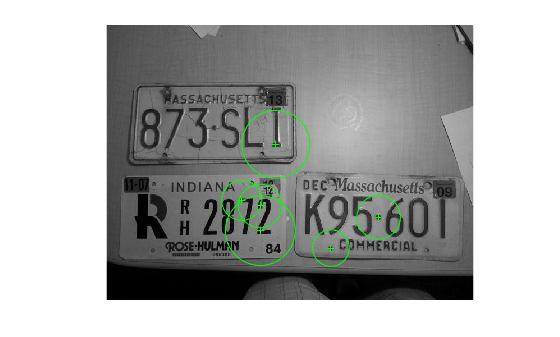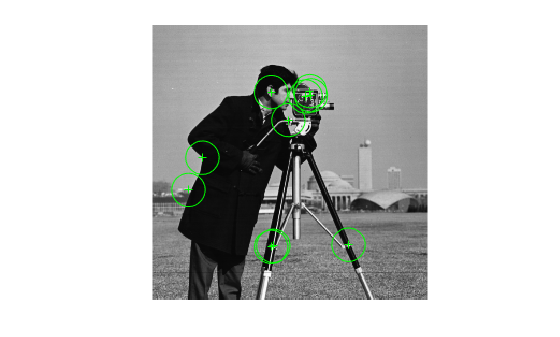# ORBPoints

Object for storing ORB keypoints

## Description

An `ORBPoints` object stores the Oriented FAST and rotated BRIEF (ORB) keypoints in an image. You can specify the keypoints and store them as an `ORBPoints` object. You can also use the `detectORBFeatures` function to detect the ORB keypoints in an image. The `detectORBFeatures` function stores the detected ORB keypoints as an `ORBPoints` object. Use Object Functions to plot, select, and manipulate the detected ORB keypoints.

## Creation

### Syntax

``points = ORBPoints``
``points = ORBPoints(location)``
``points = ORBPoints(location,Name,Value)``

### Description

````points = ORBPoints` creates an `ORBPoints` object with default property values.```

example

````points = ORBPoints(location)` creates an `ORBPoints` object from a set of location coordinates specified by `location`. The location input sets the `Location` property.```

example

````points = ORBPoints(location,Name,Value)` sets properties of the object using one or more name-value pair arguments. Enclose each property name in quotes. For example, `ORBPoints(location,'Count',15)` creates an `ORBPoints` object with `Count` property set to 15.```

## Properties

expand all

Location of keypoints, specified as an M-by-2 matrix. Each row is of the form [x y] and represents the location of a keypoint. M is the number of keypoints. You cannot set this property as a name-value pair. Use the `location` input argument.

Strength of keypoints, specified as one of these values:

• A scalar — Detected keypoints have the same strength. In this case, the object assigns the same `Metric` value to all keypoints.

• An M-element vector — Detected keypoints have different strength values. In this case, the object assigns different `Metric` value to each keypoint. M is the number of keypoints.

Example: `ORBPoints(location,'Metric',0.5)`

Data Types: `single`

Number of keypoints held by the object, specified as a nonnegative integer.

Scale factor, specified as one of these values:

• A scalar — All keypoints are detected at the same level of decomposition. In this case, the object assigns the same `Scale` value to all keypoints.

• An M-element vector — The keypoints are detected at different levels of decomposition. In this case, the object assigns different `Scale` value to each keypoint. M is the number of keypoints.

The scale factor specifies the level of decomposition at which a keypoint is detected.

Example: `ORBPoints(location,'Scale',1.2)`

Data Types: `single`

Angle of keypoints in radians, specified as one of these values:

• A scalar — Detected keypoints are of the same orientation. In this case, the object assigns the same `Orientation` value to all keypoints.

• An M-element vector — Detected keypoints are of different orientation. In this case, the object assigns different `Orientation` value to each keypoint. M is the number of keypoints.

The angle made by a keypoint is defined with reference to the horizontal axis of the image. The coordinate of the keypoint is set as the origin of the axis.

Example: `ORBPoints(location,'Orientation',0.7854)`

Data Types: `single` | `double` | `int8` | `int16` | `int32` | `int64` | `uint8` | `uint16` | `uint32` | `uint64`

## Object Functions

 `isempty` Determine if points object is empty `length` Number of stored points `plot` Plot points `selectStrongest` Select points with strongest metrics `size` Return size of points object `selectUniform` Select uniformly distributed subset of feature points

## Examples

collapse all

Read an image into the workspace.

`I = imread('licensePlates.jpg');`

Convert the image into a grayscale image.

`I = im2gray(I);`

Specify the location of keypoints in the image.

```location = [400 398; ... 485 343; ... 274 323; ... 274 367; ... 241 313; ... 302 213]; ```

Create an `ORBPoints` object and display its properties.

`points = ORBPoints(location)`
```points = 6x1 ORBPoints array with properties: Location: [6x2 single] Metric: [6x1 single] Count: 6 Scale: [6x1 single] Orientation: [6x1 single] ```

Inspect the `Scale` and `Orientation` properties of the `ORBPoints` object.

`points.Scale`
```ans = 6x1 single column vector 1 1 1 1 1 1 ```
`points.Orientation`
```ans = 6x1 single column vector 0 0 0 0 0 0 ```

Specify the scale value for each keypoint.

`scale = [2.1 2.5 2.5 4 2.3 3.9];`

Specify the angle values for the keypoints as 0.7854 radians.

`angle = 0.7854;`

Create an `ORBPoints` object with the keypoints and the updated scale and angle values. The output is an `ORBPoints` object containing keypoints with the modified `Scale` and `Orientation` properties.

`points = ORBPoints(location,'Scale',scale,'Orientation',angle);`

Inspect the updated `Scale` values.

`points.Scale`
```ans = 6x1 single column vector 2.1000 2.5000 2.5000 4.0000 2.3000 3.9000 ```

Inspect the updated `Orientation` values. Since the `Orientation` value is a scalar, the object assigns same value to all keypoints.

`points.Orientation`
```ans = 6x1 single column vector 0.7854 0.7854 0.7854 0.7854 0.7854 0.7854 ```

Display the image and plot the ORB keypoints on the image.

```figure imshow(I) hold on plot(points)```Read an image into the workspace.

`I = imread('cameraman.tif');`

Use the `detectORBFeatures` function to detect ORB keypoints in the image. The function returns the detected ORB keypoints as an `ORBPoints` object.

`points = detectORBFeatures(I);`

Use the `selectUniform` object function to select 10 ORB keypoints. The output of the `selectUniform` object function is an `ORBPoints` object.

`newPoints = selectUniform(points,10,size(I))`
```newPoints = 10x1 ORBPoints array with properties: Location: [10x2 single] Metric: [10x1 single] Count: 10 Scale: [10x1 single] Orientation: [10x1 single] ```

Display the location and scale of the selected keypoints on the image.

```figure imshow(I) hold on plot(newPoints)```Display the (x, y) coordinates of the selected keypoints.

```x = newPoints.Location(:,1); y = newPoints.Location(:,2); [x y]```
```ans = 10x2 single matrix 147 62 111 63 143 67 127 89 47 124 34 154 183 205 113 206 111 207 148 66 ```

Display the orientation of the selected keypoints.

`newPoints.Orientation`
```ans = 10x1 single column vector 5.4682 0.7888 5.3084 6.2443 2.8221 3.8440 6.1212 6.0344 4.8840 5.5535 ```

## Tips

Although `ORBPoints` can hold many points, it is a scalar object. Therefore, `numel(ORBPoints)` always returns 1. This value can differ from `length(ORBPoints)`, which returns the true number of points held by the object.

 Rublee, E., V. Rabaud, K. Konolige, and G. Bradski. "ORB: An Efficient Alternative to SIFT or SURF." In Proceedings of the 2011 International Conference on Computer Vision, pp. 2564–2571. Barcelona, Spain: IEEE, 2011.